# Comparative Design of Staircase Using StaadPro and Manual Calculations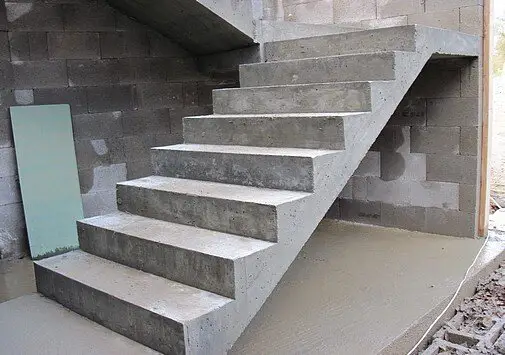Staircases provide simple solutions for vertical circulation in a building. In this post, we are going to model a simple  staircase using finite element plates, and compare the answer with manual calculations in which we assume the staircase to be a simply supported beam. The aim of this post is to verify the results obtained from the different procedures, and give reinforced concrete designers a little idea about the results to expect from their assumptions.

Let us consider a section of a staircase with dimensions as shown below;

Ultimate load on the flight = 15.719 kN/m2
Ultimate load on the landing = 14.370 kN/m2
Width of staircase = 1000 mm

Analysis Using Staad Pro (3D Finite Element Plate Model)

The 3D Model of the staircase is as given below;Fig 3: Rendered 3D Model of the Staircase

(1) Modelling the staircase as 3D Plate with pinned-pinned supports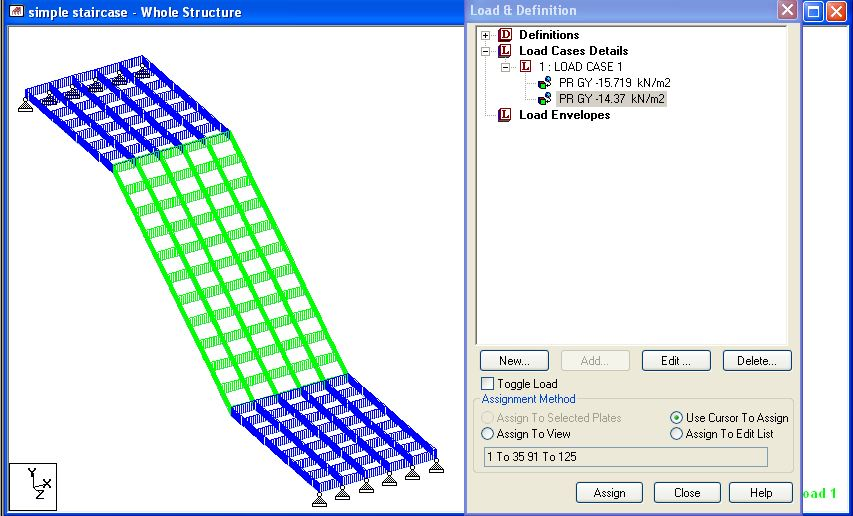Fig 4: Model of the staircase with pinned-pinned support

The static analysis of the structure gave the result below;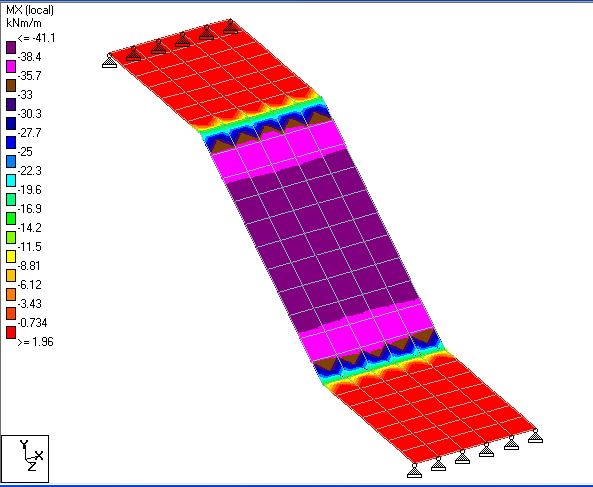Fig 5: Transverse Bending Moment on the Staircase (Mx)Fig 6: Longitudinal Bending Moment (My) on the Staircase

(2) Modelling the Staircase as 3D plate with pinned-roller support;Fig 7: Modelling the Staircase with Pinned-Roller Support

The analysis result gave the following;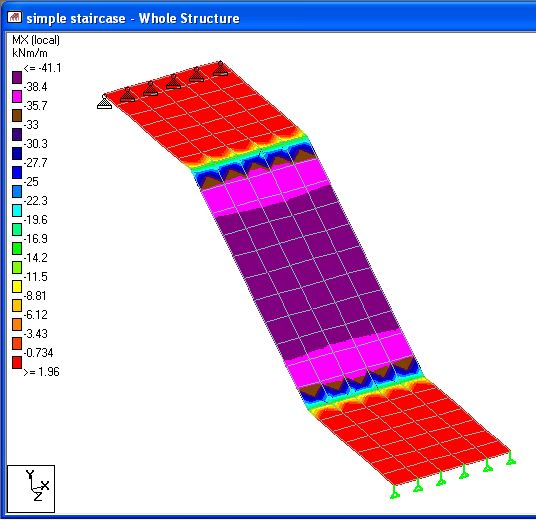Fig 8: Transverse Bending Moment (Mx)Fig 9: Longitudinal Bending Moment (My)

(3) Modelling the staircase as a static 2D frame with pinned-pinned support;

The result is as given below;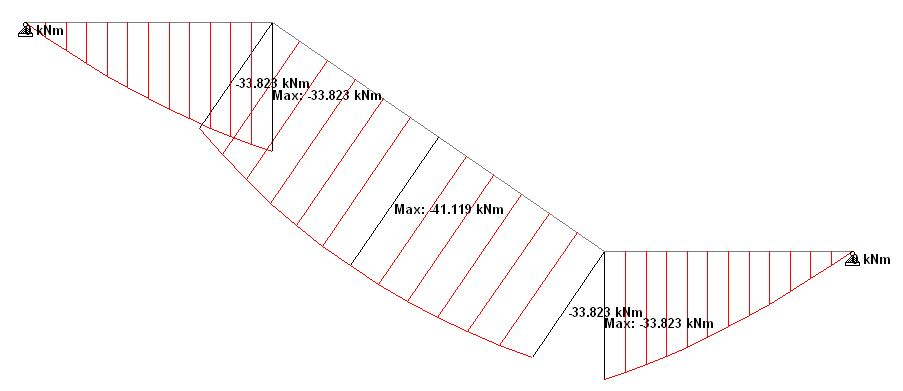Fig 10: Bending moment on the Staircase (Frame Model; pinned-pinned support)

(4) Modelling the staircase as a 2D frame with pinned-roller supportFig 11: Bending Moment on the staircase (pinned-roller model)

(5) Modelling the staircase as a simply supported beamFigure 12: Bending Moment on the stair (simply supported beam model – horizontal length)

Discussion of Results
(1) The analysis results using  the above named methods gave all the bending moments in the staircase to be sagging.
(2) Finite element plate model gave the maximum sagging at the flight to be 41.1 kNm (see Fig 5), while the maximum sagging moment on the landing was found to be 32.6 kNm (see Fig 6). This result was found in both pinned-pinned model and pinned-roller model, therefore, there is no difference in using any of the support conditions in Staad Pro.
(3) Static 2D frame model of the staircase gave the maximum sagging moment on the flight to be 41.1 kNm, while the maximum sagging moment on the flight was 33.82 kNm (see Fig 10 and 11). In principle, it can be said there is no difference in modelling the staircase as a simple 2D frame or as a 3D plate model. The former is more economical time wise.
(4) The model of span dimension adopted for the manual equivalent beam calculation was picked from SCALE software, and the maximum moment on the flight was found to be 36.04 kNm (see Fig 12). This is about 12.3% less than other models. A little consideration will show that this effect stems from the span dimensions.

To take care of this, let us modify the flight dimensions as follows;
Lf,m = Lf/cos ϕ
Where Lf is the horizontal span of flight,  Lf,m is the actual length of the flight, and ϕ is the angle of inclination of the flight.

Thus; Lf,m = 1.75m/cos 34.4 = 2.12m

This gave the result below;Fig 13: Bending moment on the stair (simply supported beam model, developed length)

We can see that is more comparable and conservative when compared with results from computer models. Therefore, for all simple staircases, we can use the developed length of the flight instead of the horizontal length to analyse the staircase as a simply supported beam. However when checking for deflection, we should use the horizontal length.

Thank you for visiting Structville today. God bless.

1.Anonymous

Erroneous conclusions. The static 2D frame model is wrong, the dead load should be multiplied times the Cos of the angle, and live load should be multiplied times the square Cos of the angle.

2. Well, the post is not about load analysis, but modelling and structural analysis. However, if you can provide more insight on the point you are trying to make, it will be deeply appreciated. Thanks.

3.Anonymous

It's obvious that you cannot apply the full load at the inclined portion of the stair, but just the component perpendicular to the surface. Since the live load is applied to the horizontal projection, the component is squared. Never ever use the horizontal length of the stair, as you incorrectly concluded.

4. Ok. Let there be no confusion. The global load on the staircase (both landing and flight) has been determined prior to this post, and it is not the subject matter. In the post, the conclusion was NEVER that you should use horizontal length while using simply supported beam model (read the discussions very well). However, what I said was that while checking for deflection (L/d ratio in this case), horizontal length can be used.

5.Anonymous

That's incorrect. Do not use use the horizontal length to check the deflections either.

6. Anonymous, please kindly design yours let us all learn

7. Your article was of great use! Thanks to it, I could find and choose the right materials. website

8. Programmable calculators were later invented to make complicated calculations. The scientific calculators that we see today are latest inventions that came about in these segments.999 meaning in numerology